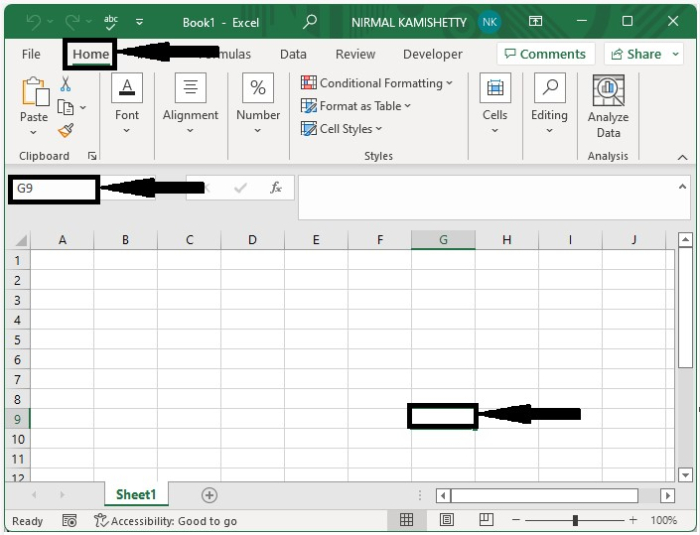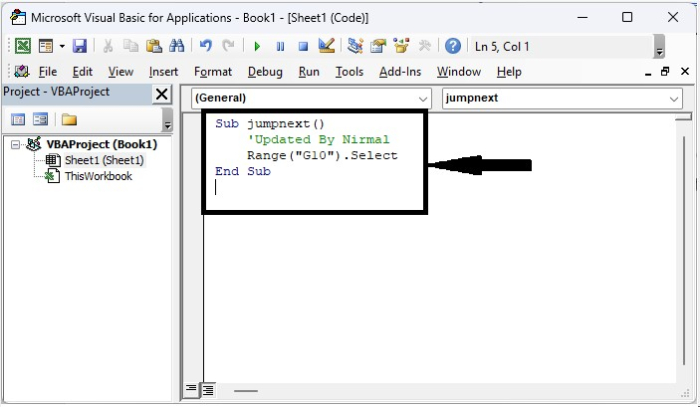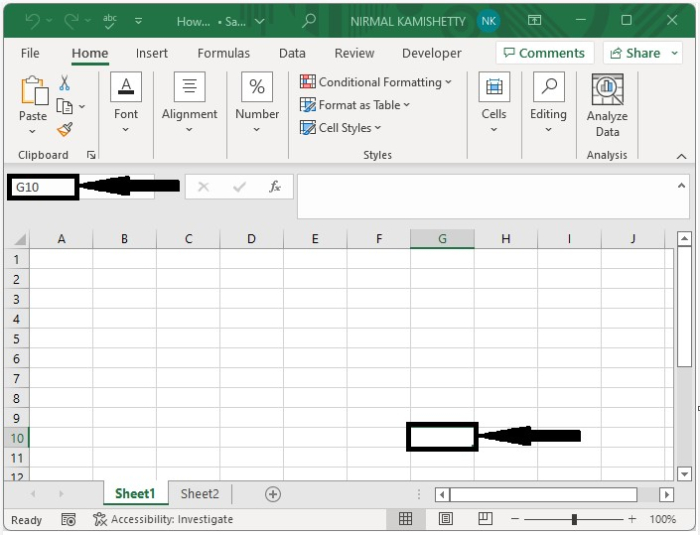# How to Automatically Move the Cursor to a Specific Cell in Excel?

When we are dealing with large amounts of data moving around, accessing the data is one of the most often applied functions in Excel. Finding the data set could be a problem because the large dataset can consume a lot of time. Even when we know the address of the cell, it will take a lot of work to find it. We solve this problem by automatically moving the cursor to a specific cell. This tutorial will help you understand how we can automatically move the cursor to a specific cell in Excel. This process can be done in two ways: first, by using the name box, and second, by using the VBA application.

## Automatically Move the Cursor to a Specific Cell Using Name Box

Here we will use the name box. Let us see a straightforward process to know how we can automatically move the cursor to a specific cell using the name box in Excel.

It is a fairly straightforward process. Click on the name box, enter the cell address you want to navigate to, and click on Enter to get the result.## Automatically Move the Cursor to a Specific Cell Using VBA

Here we insert a VBA module with a cell address, then run the module to go to the cell location. Let us see an effortless process to know how we can automatically move the cursor to a specific cell in Excel.

Step 1

Let us consider a new Excel sheet, then right-click on the sheet name and select view code to open the vba application, and enter the programme into the text box as shown in the below image.

### Example

Sub jumpnext()
'Updated By Nirmal
Range("G10").Select
End Sub


In the code, G10 is the address of cell where we want to move.Step 2

Now save the sheet as a VBA-enabled workbook and click on F5 to run the code, and our process will be completed as shown in the below image.## Conclusion

In this tutorial, we used a simple example to demonstrate how we can automatically move the cursor to a specific cell in Excel.